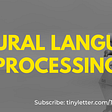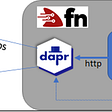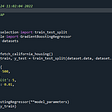# Artificial Intelligence & Neural Networks

Click To Get The Source Code In C#

Introduction to Genetic Algorithm

A genetic algorithm is a search heuristic algorithm that reflects the cognitive operation of natural selection where the best people are chosen for reproduction in society to produce offspring or children of the next generation. This algorithm can be applied to solve constrained and unconstrained problems using the hypothesis of natural selection. The genetic algorithm works on the mechanics of biological development. On the basis of the evolutionary process and artificial intelligence, genetic algorithms provide computer systems with a method of problem-solving.

In every human being, chromosomes store the transmitted data. Each genetic information is called the genome. Same as, in computer programs, a set of variables stores information based on the genomes. It can be bits, strings, etc. These digital chromosomes represent the data that can be utilized to procreate the next genomes.

Advantages or Purpose of Genetic Algorithm

· Genetic algorithm provides the best-optimized solution to every problem.

· It provides efficient and effective techniques for machine learning applications.

· As it is based on artificial intelligence and machine learning, it is widely used in businesses, engineering circles, and scientific research purposes..

· It’s an abstraction of real biological evolution.

· It is applied to resolve complex problems.

· Primarily focus on optimization.

Best Idea

“Select the best, discard the rest.”

It means that we select the best genes that can be used to produce the next generation. And the genes that are not able to produce the next generation, are discarded.

Applications of Genetic Algorithm

There are many applications of genetic algorithms, some of them are given below:

· Travelling salesman problem

· Image processing

· Game theory equilibrium

· Bioinformatics

· Building phylogenetic trees

· Quality control

· Datacenter/server farm

· Neural Networks

· Knapsack Problem

Components or Phases of Genetic Algorithm

We will explain all the phases of the genetic algorithm by using an example of “Knapsack Problem using Genetic Algorithm”

Knapsack Problem

In combinational optimization, there is a problem called Knapsack Problem. In this problem, a set of items is given along with their weights and values. On the basis of a given set of items, the total weight should be less than the maximum weight capacity of the Knapsack.

The knapsack problem can be solved by using different methods of computational algorithms. But here we will solve this problem by using a genetic algorithm. So, we could put valuable items in the knapsack.

Example:

Let us consider, we have four items and their weights and values are given: in the below table:

Now, we have to put all these items in a bag.

Let,

Maximum Capacity in Knapsack = 12 kg

Note that, total weight should be less than the maximum weight of the knapsack.

A, B, C, and D are representing the item names. We can also select real-world items like fruits, vegetables, groceries, etc.

Step 1: Chromosome Encoding / Initial Population

In chromosome encoding, we randomly generate an initial population of given items. In each chromosome, bits (0s & 1s) are generated randomly and each bit will represent a gene. And a set of all chromosomes will represent a population of the current generation. For example, consider a sample of population, chromosome, and gene for understanding:

Here,

0 = represents the absence of an item in the knapsack

1 = represents the presence of an item in the knapsack

Now, we will generate the initial population for the given set of items,

Here Pi is the two-dimensional array that represents the given items’ chromosomes and the genotypes.

As,

Number of items = 4
Total set space = 2⁴ = 16

Here, total set space means there will be 16 numbers genes and there will be 4 chromosomes as given below:

Generation: 1

So, the initial population is generated randomly and the genes (0’s and 1’s) are also generated randomly.

Step 2: Fitness Function

The fitness function determines the ability of each individual to compete with other individuals. The individual whose fitness score is greater and weight is less than the maximum capacity of knapsack will be accepted and those whose weight is equal or greater than the maximum weight will be discarded.

Now let us calculate the fitness value of each chromosome. Put the values and weights of those items that are showing their presence (1) in the chromosomes.

For chromosome C1

Value of Knapsack = value of B + value of C = 5 + 10
Value of knapsack for C1 = 15

Weight of knapsack = weight of B + weight of C = 3 + 7
Weight of Knapsack for C1 = 10 kg < 12 kg

Chromosome C1 is accepted

For chromosome C2

Value of Knapsack = value of B + value of D = 5 + 7
Value of knapsack for C2 = 15

Weight of knapsack = weight of B + weight of D = 3 + 2
Weight of Knapsack for C2 = 5 kg < 12 kg

Chromosome C2 is accepted

For chromosome C3

Value of Knapsack = value of A + value of B + value of D = 12 + 5 + 7
Value of knapsack for C3 = 24

Weight of knapsack = weight of A + weight of B + weight of D = 5 + 3 + 2
Weight of Knapsack for C3 = 10 kg < 12 kg

Chromosome C3 is accepted

For chromosome C4

Value of Knapsack = value of A + value of B + value of C + value of D
Value of Knapsack = 12 + 5 + 10 + 7
Value of knapsack for C4 = 34

Weight of knapsack = weight of A + weight of B + weight of C + weight of D
Weight of knapsack = 5 + 3 + 7 + 2
Weight of Knapsack for C4 = 17 kg > 12 kg

So, from the above calculation, C4 is discarded because its weight is larger than the maximum weight of the Knapsack.

Hence the final solutions are:

Fitness value of C1 = 15
Fitness value of C2 = 12
Fitness value of C3 = 24
Fitness value of C4 = 0

Note: We take the fitness value of C4 as zero because it has been discarded, so there will be no effect on genotypes if we use it. Hence it is not fitter than others, it’s better to take its value as zero.

Step 3: Selection

In the selection process, the fittest individual will be selected that will be used to reproduce the next generation. On the basis of the fitness score, two parents will selected.

Total fitness value = 15 + 12 + 24 + 0
Total fitness value = 51
Largest fitness value is of C3 = 24

The individual(s) that has the highest fitness value will be able to produce next generation. The selection can be placed by different methods; like a roulette wheel, etc.

Probability

Now find the probability of each chromosome by using:

Probability of Ci = Fitness value of Ci / Total Fitness value

Find out how much space each chromosome occupies;

Probability of C1 = 15 / 51
Probability of C2 = 12 / 51
Probability of C3 = 24 / 51
Probability of C4 = 0

Hence, from the above probability calculations;

C3 will occupy 24/51 of the wheel and has a high chance of using it.

C4 has a 0% chance of using.

Roulette Wheel

Spin the roulette wheel and whenever the wheel stops, the individual gets selected at that point.

The individual that has the highest fitness value gets a larger size of the wheel.

From the above observations roulette wheel will be:

Let us consider, after spinning the Roulette wheel, in 1st spin C3 is selected and then C1 after that C3 and C2. Individuals of next-generation are selected as follows:

Generation: 2

Now, here the parents are C3 and C1

These parents will be used to produce further child/offspring.

Step 4: Crossover

Take the selected parent's chromosomes for making and mixing the genetic material to produce a child or offspring.

One-point Crossover

Randomly select the position on the chromosomes about which gene would be exchanged. Consider the selected crossover points:

Now, the crossover points are marked and the child/offspring will be produced as:

Child / Offspring (OS)

After crossover, offspring will be replaced by chromosomes 1 and 2 and the next generation will be:

Generation: 3

Step 5: Mutation

Introduce the diversity within the population so that the search algorithm doesn’t necessarily get stuck at local maxima. Let us consider, chromosome C which will be selected randomly from the population, now randomly select a gene from C.

A flip happens at the selected genes and zero becomes one and one becomes zero.

Chromosome C after mutation will become C’

Termination

If the algorithm doesn’t produce offspring, it means the genetic algorithm has provided an optimized solution

--

--

--

Computer Scientist, Content Creator, Video Producer. I create content that erects a positive hope with some taste of entertainment.

Love podcasts or audiobooks? Learn on the go with our new app.

## TICKET FILTER## Object Detection with Yolov5## Importance of NLP in Data Science## Breast Cancer prediction Model## A Practical Dive into Data Fusion For Self-Driving Cars## The whiteboard analogy to deal vanishing and exploding gradients## Batch Normalization## Machine Learning (Classification) Intro Tutorial## Muzamil Shahbaz

Computer Scientist, Content Creator, Video Producer. I create content that erects a positive hope with some taste of entertainment.

## Witness the power of Intel® iGPU with Azure IoT Edge for Linux on Windows(EFLOW) & OpenVINO™…## Marrying Fn Functions to Dapr.io-leverage the power of the proxy## Best Way to Deploy Sci-kit Learn model in dot Net Application